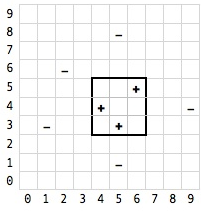2 views

The FIND-S algorithm is probably one of the most simple machine learning algorithms. However, I can't find many examples out there. Just the standard 'sunny, rainy, play-ball' examples that are always used in machine learning. Please, could someone help me with this application (its a past exam question in machine learning)?

Hypotheses are of the form a <= x <= b, c <= y <= d where x and y are points in an x,y plane and c and d are an integer. Basically, these hypotheses define rectangles in the x,y space.

These are the training examples where - is a negative example and + is a positive example and the pairs are the x,y coordinates:

+ 4, 4

+ 5, 3

+ 6, 5

- 1, 3

- 2, 6

- 5, 1

- 5, 8

- 9, 4

All I want to do is apply FIND-S to this example! It must be simple! Either some tips or a solution would be awesome.

Thank you.

by (108k points)

Find-S seeks the most specific hypothesis that fits all the positive examples (negatives are ignored).

In your case, there is a graphical interpretation: "find the smallest rectangle that contains all the '+' coordinates".which would be a=4, b=6, c=3, d=5.

You can refer the following algorithm:

Define a hypothesis rectangle h[a,b,c,d], and initialise it to [-,-,-,-]

for each + example e

if e is not within h

enlarge h to be just big enough to hold e (and all previous e's)

} else { do nothing: h already contains e } }

If we step through this with your training set, we get:

0. h = [-,-,-,-] // initial value

1. h = [4,4,4,4] // (4,4) is not in h: change h so it just contains (4,4)

2. h = [4,5,3,4] // (5,3) is not in h, so enlarge h to fit (4,4) and (5,3)

3. h = [4,6,3,5] // (6,5) is not in h, so enlarge again

4. // no more positive examples left, so we're done.